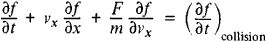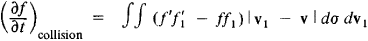# Kinetic

(redirected from -kinetic)
Also found in: Dictionary, Thesaurus, Medical.

## kinetic

[kə′ned·ik]
(science and technology)
Pertaining to or producing motion.
McGraw-Hill Dictionary of Scientific & Technical Terms, 6E, Copyright © 2003 by The McGraw-Hill Companies, Inc.
The following article is from The Great Soviet Encyclopedia (1979). It might be outdated or ideologically biased.

## Boltzmann (Kinetic) Equation

an equation for the distribution function f(v, r, t) of gas molecules in the velocities ν and coordinates r (as functions of time t) that describes nonequilibrium processes in gases of low density. The function f determines the average number of particles having velocities within a small range from ν to ν + Δν and coordinates within a small range from r to r + Δr. If the distribution function depends only on the coordinate x and the velocity component vx, then the Boltzmann (kinetic) equation has the formwhere m is the mass of the particle. The rate of change of the distribution function is characterized by the partial derivative ∂f/∂t. The second term in the equation, which is proportional to the partial derivative of the distribution function with respect to the coordinate, takes account of the change in f as a result of the movement of particles in space. The third term determines the change in the distribution function owing to the action of external forces F. The term on the right-hand side of the equation, which characterizes the rate of change of the distribution function owing to collisions between particles, depends on f and the nature of the forces of interaction between particles and is equal toHere, f, f1, and fʹ, f1ʹ are the distribution functions of the molecules before and after collision, respectively; v, v1 are the velocities of the molecules before collision; and dσ =σdΩ is the differential effective scattering cross section into the solid angle dΩ (in the laboratory coordinate system) dependent on the law of molecular interaction. When molecules are simulated by rigid elastic spheres (of radius R), σ = 4R2 cos θ, where θ is the angle between the relative velocity v1 - v of the colliding molecules and the line connecting its centers. The kinetic equation was derived by L. Boltzmann in 1872.

Various generalizations of the Boltzmann kinetic equation describe the behavior of electron gas in metals, of phonons in crystal lattices, and so forth (however, these equations are often called simply kinetic equations, or transport equations).

G. IA. MIAKISHEV